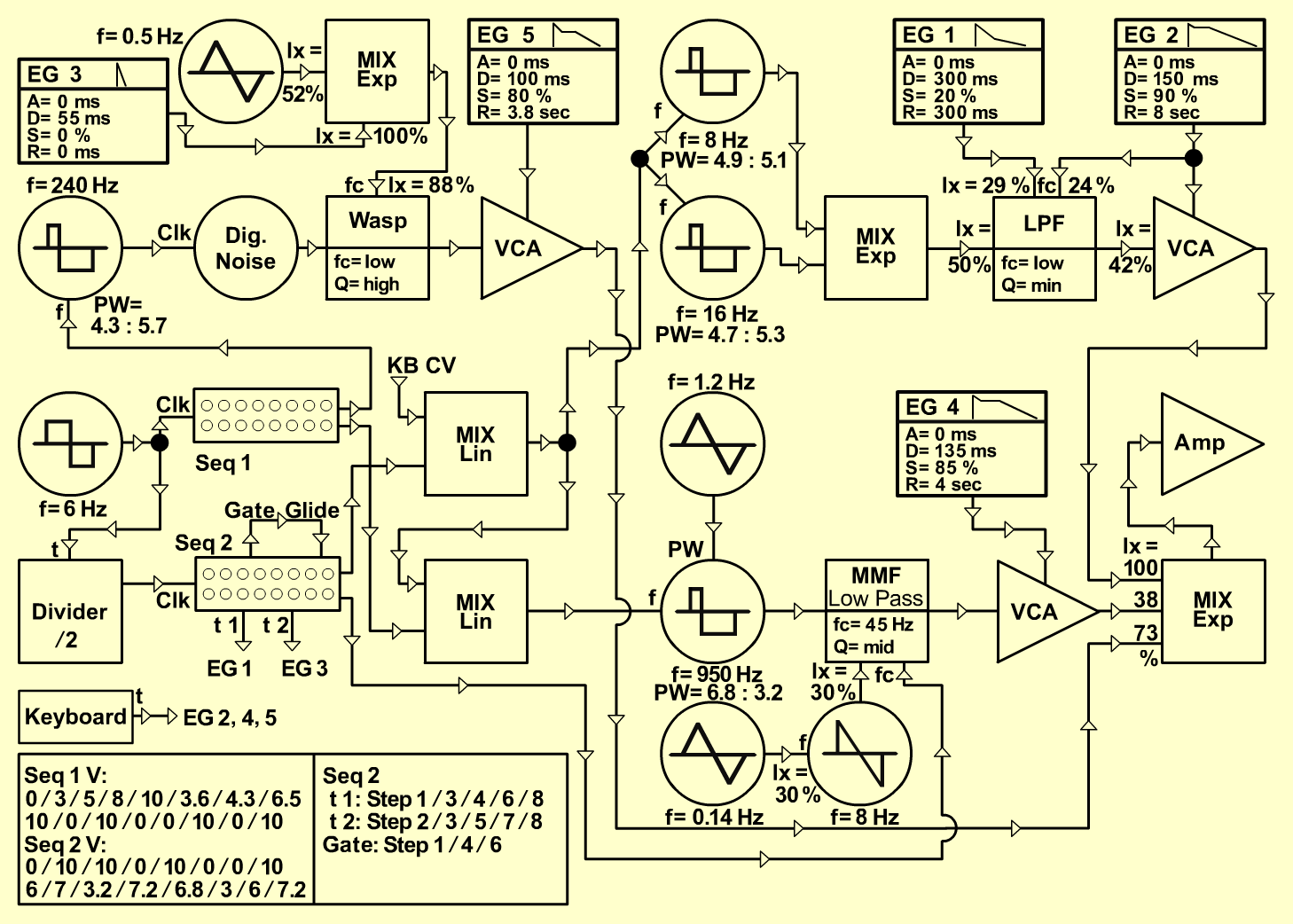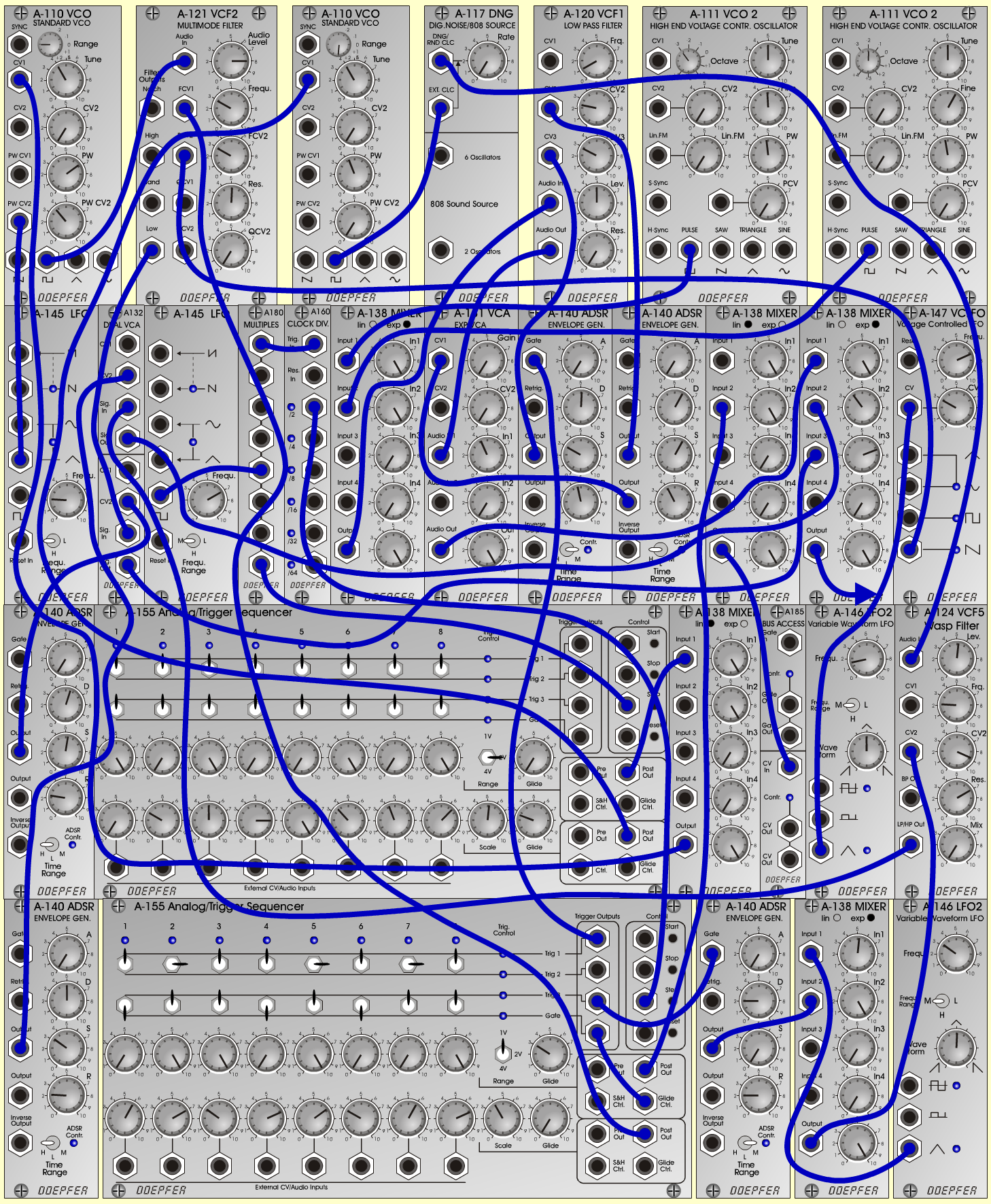ComplexSeqTextureD o e p f e r A - 100 Connections: Settings: A-110/1 (Pulse)  <=>  A-121 (Audio In) A-110/2 (Pulse)  <=>  A-117 (Ext Clk) A-138/1 (Output)  <=>  A-120 (Audio In) A-120 (Audio Out)  <=>  A-131/Vers. 2 (Audio In 1) A-140/1 (Output)  <=>  A-120 (CV3) A-140/2 (Output)  <=>  A-120 (CV2) A-140/2 (Output)  <=>  A-131/V.2 (CV1) A-145/1 (Pulse)  <=>  A-180 A-180  <=>  A-155/1 (Step/Clock) A-180  <=>  A-160 (Trig In) A-160 (/2)  <=>  A-155/2 (Step/Clock) A-145 (Tri)  <=>  A-110 (PWCV2) A-121 (Low)  <=>  A-132 (Lower Sig In) A-138lin/1 (Output)  <=>  A-185 (CV In) A-185 (Bus) <=>  A-111/1 (CV1) A-185 (Bus) <=>  A-111/2 (CV1) A-185 (CV Out)  <=>  A-138lin/2 (Input 2) A-155/1 (Upper Post Out)  <=>  A-138lin/2 (Input 1) A-155/1 (Lower Post Out)  <=>  A-110/2 (VC 1) A-138lin/2 (Output)  <=>  A-110/1 (VC 1) A-155/2 (Upper Post Out)  <=>  A-138lin/1 (Input 2) A-155/2 (Lower Post Out)  <=>  A-121 (FCV 1) A-155/2 (Gate)  <=>  A-155/2 (Upper Glide Ctrl) A-155/2 (Trig 1)  <=>  A-140/1 (Gate) A-155/2 (Trig 3)  <=>  A-140/3 (Gate) A-147 (Saw)  <=>  A-121 (FCV 2) A-111/1 (Pulse)  <=>  A-138exp/1 (Input 1) A-111/2 (Pulse)  <=>  A-138exp/1 (Input 2) A-138exp/2 (Output)  <=> Amplifier A-117 (DNG)  <=>  A-124 (Audio In) A-140/3 (Output)  <=> A-138exp/3 (Input 2) A-140/4 (Output)  <=>  A-132 (Lower CV 2) A-138exp/3 (Output)  <=>  A-124 (CV2) A-124 (LP/HP Out)  <=>  A-132 (Upper Sig. In) A-131/V.2 (Audio Out)  <=>  A-138exp/2 (Input 1) A-140/5 (Output)  <=>  A-132 (Upper CV2) A-146/1 (Triangle)  <=>  A-147 (CV) A-146/2 (Triangle)  <=>  A-138exp/3 (Input 1) A-132 (Upper Sig Out)  <=>  A-138exp/2 (Input 2) A-132 (Lower Sig Out)  <=>  A-138exp/2 (Input 3) KB CV Out  <=>  A-138lin/1 (Input 1) KB Gate Out  <=>  A-140/2 (Gate) KB Gate Out  <=>  A-140/4 (Gate) KB Gate Out  <=>  A-140/5 (Gate) A-110 (Range = 5, Tune = 4,  PW = 6.8, PW CV 2 = 3.7) A-110 (Range = 3, Tune = 4,  PW = 4.3) A-138exp/1 (In 1 = 10, In 2 = 10, Out = 10) A-120 (Freq = 1, CV2 = 2.4, CV3 = 2.9, Lev = 5, Res = 0) A-140/1 (A = 0, D = 5.1, S = 3, R = 4.6, Range = M) A-140/2 (A = 0, D = 6, S = 6, R = 4.1, Range = H) A-145/1 (Frq = 7, Range = M) A-145/2 (Frq = 2.1, Range = M) A-121 (Audio Level = 8, Freq = 3, FCV 2 = 3, Res = 5.1) A-138lin/1 (In 1 = 10, In 2 = 10, Out = 10) A-155/1 (Row 1: 1 = 10, 2 = 0, 3 = 10, 4 = 0, 5 = 0, 6 = 10, 7 = 0, 8 = 10, Range = 2V, Glide = 0; Row 2: 1 = 0, 2 = 3, 3 = 5, 4 = 8, 5 = 10, 6 = 3.6, 7 = 4.3, 8 = 6.5, Scale = 5.2, Glide = 2.7) A-138lin/2 (In 1 = 10, In 2 = 10, Out = 10) A-155/2 (Row 1: 1 = 0, 2 = 10, 3 = 10, 4 = 0, 5 = 10, 6 = 0, 7 = 0, 8 = 10, Range = 1V, Glide = 3.1; Row 2: 1 = 6, 2 = 7, 3 = 3.2, 4 = 7.2, 5 = 6.8, 6 = 3, 7 = 6, 8 = 7.2, Scale = 4, Glide = 0; Trig 1: 1, 3, 4, 6, 8; Trig 3: 2, 3, 5, 7, 8; Gate: 1, 4, 6) A-147 (Freq = 7.2, CV = 3) A-111/1 (Octave = -3, Tune = 5, Fine = 4.9, PW = 4.8) A-111/2 (Octave = -2, Tune = 5, Fine = 6, PW = 4.7) A-138exp/2 (In 1 = 10, In 2 = 3.8, In 3 = 7.3, Out = 10) A-140/3 (A = 0, D = 2, S = 0, R = 2, Range = M) A-140/4 (A = 0, D = 5.6, S = 5.3, R = 2.3, Range = H) A-138exp/3 (In 1 = 5.2, In 2 = 10, Out = 10) A-124 (Lev = 5.2, Frq = 2.1, CV 2 8.8, Res = 7, Mix = 0) A-131 (Gain = 0, Audio In 1 = 4.2,  Audio Out = 10) A-140/5 (A = 0, D = 5, S = 3.2, R = 2.1, Range = H) A-146/1 (Freq = 1.6, Wave Form = 5, Range = M) A-146/2 (Freq = 3.2, Wave Form = 5.1, Range = M) Bakis Sirros, January 2002  group: PARALLEL WORLDSSound sample ComplexSeq